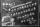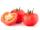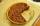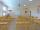How many products weighing 12.5 kg can be loaded on a cargo car with a load of 1.5 t to load two-thirds?

Result

n =  80

#### Solution:Leave us a comment of example and its solution (i.e. if it is still somewhat unclear...):Be the first to comment!#### To solve this example are needed these knowledge from mathematics:

Need help calculate sum, simplify or multiply fractions? Try our fraction calculator.

## Next similar examples:

1. Fractions 4How many 2/3s are in 6?
2. Lengths of the poolMiguel swam 6 lengths of the pool. Mat swam 3 times as far as Miguel. Lionel swam 1/3 as far as Miguel. How many lengths did mat swim?
3. Scouts 44/7 of the students in a school are boys. If 3/8 of the boys are scouts, how many scouts are there in a school of 1878 students?
4. Farmers 2On Wednesday the farmers at the Grant Farm picked 2 barrels of tomatoes. Thursday, the farmers picked 1/2 as many tomatoes as on Wednesday. How many barrels of tomatoes did the farmers pick on Thursday?
5. Product of two fractionsProduct of two fractions is 9 3/5 . If one of the fraction is 9 3/7. Find the other fraction.
6. Quotient 3If the quotient of 8/13 and 2 is subtracted from the product of 1 3/4 and 8/21, what is the difference?
7. One-third 2One-third of the people in a barangay petitioned the council to allow them to plant in vacant lots and another 1/5 of the people petitioned to have a regular garbage collection. What FRACTION of the barangay population made the petition?
8. Fractions 3Calculate 1/9 of 27:
9. Pizza 5You have 2/4 of a pizza and you want to share it equally between 2 people how much pizza does each person get?
10. In fractionsAn ant climbs 2/5 of the pole on the first hour and climbs 1/4 of the pole on the next hour. What part of the pole does the ant climb in two hours?
11. Pie IIVili ate three pieces of pie. If it pieces is 1/8 how much pie did he eat?
12. Fraction to decimalWrite the fraction 3/22 as a decimal.
13. Mixed2improperWrite the mixed number as an improper fraction. 166 2/3
14. Classroom 4In a class of 36 pupils, 2/3 are girls. How much it is in a class girls and boys?
15. PieVili ate three pieces of pie . If it piece is 1/8 how much pie did he eat?
16. ZdeněkZdeněk picked up 15 l of water from a 100-liter full-water barrel. Write a fraction of what part of Zdeněk's water he picked.
17. Fraction and a decimalWrite as a fraction and a decimal. One and two plus three and five hundredths